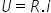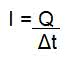Definitions

# Electric Current

Electric current designates the ordered movement of electric charges (electrified particles called ions or electrons) within a conducting system.

This system presents an electric potential difference (ddp) or electric voltage.

Its flowing through resistors can transform electrical energy into thermal energy (heat), in a phenomenon known as the Joule Effect .

The resistance of a conducting wire facilitates or hinders the passage of it, being calculated through the formula of Ohm’s First Law ( R=U/I ).

Electronic devices, batteries and batteries, have a negative pole and a positive pole. This explains the potential difference (ddp) present in the circuit of each one of them.

Note that the direction of it is characterized in two ways. One of them is the “ real electric current ”, that is, the one that has the direction of the movement of the electrons.

The other way is the “ conventional electric current ”, whose direction is contrary to the movement of electrons and is marked by the movement of positive electric charges.

In the International System of Units (SI), the intensity of the electric current is measured in Ampere (A), the resistance in Ohm (Ω) and the electric voltage (ddp) is measured in Volts (V).

## Electrical Conductors

Electrical conductors are materials that allow the movement of electrons, that is, the passage of it. A material is considered an electrical conductor depending on the potential difference to which it is subjected.

The best electrical conductors are metals, on the other hand, materials that hinder the movement of electrons are called insulators. Examples are wood, plastic and paper.

There are three types of conductors :

• Solids – characterized by the movement of free electrons;
• Liquids – movement of positive and negative charges;
• Gaseous – movement of cations and anions.

## Types

• Direct Current (DC) : it has constant direction and intensity, that is, it presents a continuous potential difference (ddp), generated by cells and batteries.
• Alternating Current (AC) : it has varied direction and intensity, that is, it presents a potential difference (ddp) is alternating, generated by the power plants.

## Electric tension

Electric voltage , also called potential difference (ddp), characterizes the difference in electric potential between two points in a conductor. It is, therefore, the force resulting from the movement of electrons in a given circuit.

In the International System (SI), electrical voltage is measured in Volts (V). To calculate the voltage in an electrical circuit , use the expression:Where,

U = Electrical voltage (V)
R = Resistance (Ω)
i = Current intensity (A)

## Electric Current Intensity

The intensity of the electric current, represented by the letter ‘i’, designates the amount of electric charge (Q) that crosses a conductor in a given time interval (Δt).

In the international system, its unit of measurement is the Ampere (A), calculated using the following expression:Where,

I : current intensity (A)
Q : electric charge (C)
Δt : time interval (s)

## Electricity

Electric energy is produced from the electric potential of two points of a conductor. In this way, to calculate the electrical energy, the equation is used:

And he = P . ∆t

Where:

el : electrical energy (kWh)
P : power (kW)
∆t : time variation (h)